# AA1 – Life Sciences

## Random Bifurcations in Chemical Reaction Networks

Maximilian Engel

Project Members

Guillermo Olicón Méndez

Project Duration

01.10.2021 – 30.09.2023

Located at

FU Berlin

### Description

In this project, we develop a random bifurcation theory for chemical reaction networks whose kinetics are properly modeled by Markov jump processes. For large reactants, model reduction to reaction rate equations (ODEs) and Langevin equations (SDEs) is possible and computationally alleviating. We investigate which model reductions succeed in preserving pathwise properties of the jump process in terms of dynamical stability and bifurcation behavior stemming from varying reaction rates.

In more details, we consider general models of large sets of molecules that can be allocated to a certain number of different species. Reactions, changing the number of molecules of a subset of species, occur at certain rates  which are defined as propensity funtions involving reaction rate constants. Assuming that these functions depend only on the state of the whole reaction system, one can describe the changes of the system over time, i.e. the dynamics, as a Markov jump process driven by independent Poisson processes. For high accumulations of reaction events, the Poisson variables can be approximated by normal random variables, yielding the chemical Langevin equation (CLE) which is a stochastic differential equation (SDE). In the thermodynamic limit where volume and volume-dependent molecule numbers scale in a fixed proportion approaching infinity, we may average over the noise terms to obtain the purely deterministic version of the CLE, i.e. an ODE also called reaction rate equation (RRE).

For all three approximation levels, there are corresponding differential equations, describing the evolution of the probability distribution of states ; in the stochastic case, this evolution is described by the forward Kolmogorov equation, which takes the form of the chemical master equation for the Markov jump process and the form of a Fokker-Planck equation for the CLE. The corresponding PDE for the RRE is called Liouville equation. Many investigations focus on these equations comparing distributions of the reaction process and its approximation models, and by that only capture statistical properties of the processes.

We employ the perspective of random dynamical systems (RDS)  in order to understand trajectory-wise behavior of the Markov jump process in comparison to the SDE solution, and to demonstrate what can be missed, also in the thermodynamic limit, by only focusing on expectations given by solutions of the RRE. The RDS approach compares two trajectories with the same noise realizations and analyzes whether they synchronize or diverge asymptotically, determining the form of a random attractor. By applying techniques of linearization and multiplicative ergodic theory, we are the first to investigate and compare thoroughly the stability behavior of all three models; this becomes highly relevant when changes of rate constants  are considered such that bifurcations can be detected. A crucial role is played by the Hopf bifurcation, where an attracting equilibrium loses stability and an attracting periodic orbit occurs, which exhibits complicated behavior under noise perturbations (see first Figure below).

One first project concerns the Brusselator, a chemical toy model, for which we investigate transient and asymptotic phenomena in the Langevin dynamics (see several Figures below), building up tools for new RDS descriptions of stochastic oscillators in chemical reaction networks (CRNs). A second current project investigates synchronization effects for underlying Markov jump process in CRNs, in collaboration with S. Winkelmann’s group at ZIB. Both lines of study are anticipated to lead to preprints in the first half of 2022.

Project Webpages

Selected Publications

M. Engel, J.S.W. Lamb and M. Rasmussen. Conditioned Lyapunov exponents for random dynamical systems. Transactions of the American Mathematical Society, 372(9): 6343-6370, 2019. https://doi.org/10.1090/tran/7803

M. Engel, J.S.W. Lamb and M. Rasmussen. Bifurcation analysis of a stochastically driven limit cycle. Communications in Mathematical Physics, 365: 935-942, 2019.
https://doi.org/10.1007/s00220-019-03298-7

Selected Pictures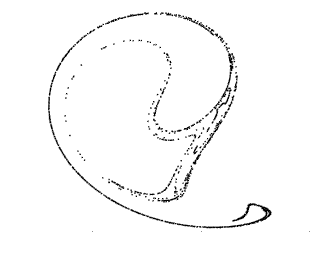##### Chaotic random attractor from a stochastic Hopf bifurcation
Such a fractal-like attractor with chaotic properties can occur in stochastically perturbed systems (CLE) that experience a Hopf bifurcation in the deterministic case (RRE), for example in a typical biological modle like the so-called Brusselator.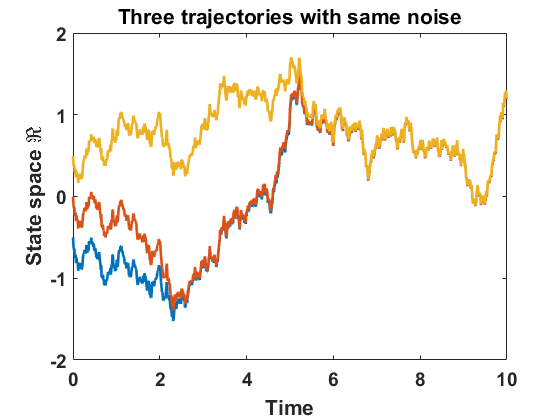##### Synchronization of stochastic trajectories driven by the same noise
Synchonization of trajectories, i.e. the random attractor shrinking to a point, is the other typical behavior of random dynamical systems, also occurring in Hopf bifurcations under the influence of noise.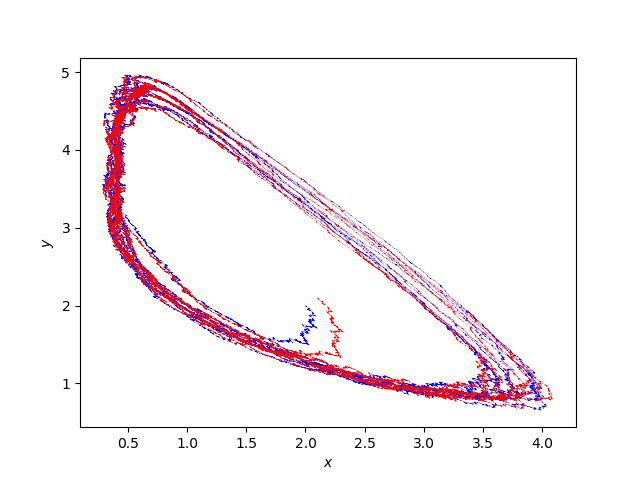##### A stochastic Brusselator

When studied from the RRE perspective (a completely deterministic point of view), the Brusselator exhibits the emergence of an attracting limit cycle for certain values of the system’s parameters.

For sufficiently large values of the system’s volume, its corresponding chemical Langevin equation shows some similarities with its deterministic counterpart. For instance, there is still an oscillatory behaviour reminiscent of the RRE formulation.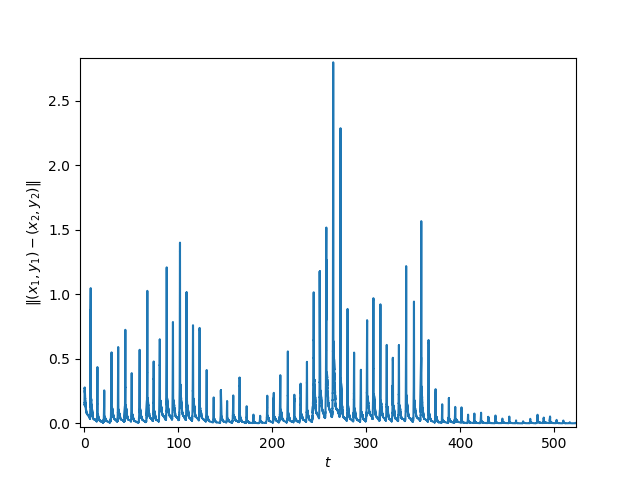##### Finite time instabilities

When studied from the RRE perspective (a completely deterministic point of view), the Brusselator exhibits the emergence of an attracting limit cycle for certain values of the system’s parameters.

For sufficiently large values of the system’s volume, its corresponding chemical Langevin equation shows some similarities with its deterministic counterpart. For instance, there is still an oscillatory behaviour reminiscent of the RRE formulation.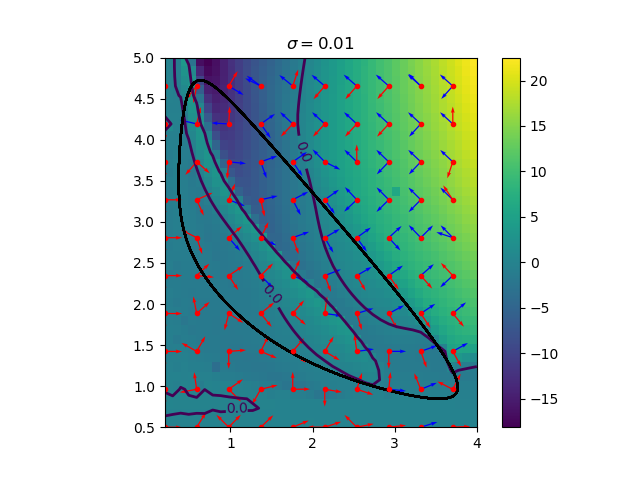##### Covariant Lyapunov vectors and Finite-Time Lyapunov exponents

Covariant Lyapunov Vectors (CLV) indicate special directions along which nearby orbits exponentially expand away or contract towards each other. The rates at which they do so in a certain time window are called Finite-Time Lyapunov Exponents (FTLE). A positive sign in FTLEs indicates local exponential deviation of nearby trajectories, while a negative sign indicates the opposite.

These tools from (random) dynamical systems have proven to be useful in all sorts of applications, including those involving real-world data. For chemical reaction networks one can exhibit a landscape of finite-time attraction and repulsion throughout the state space, which helps to understand regions of high finite-time instabilities, qualitative changes in finite-time behaviour, etc.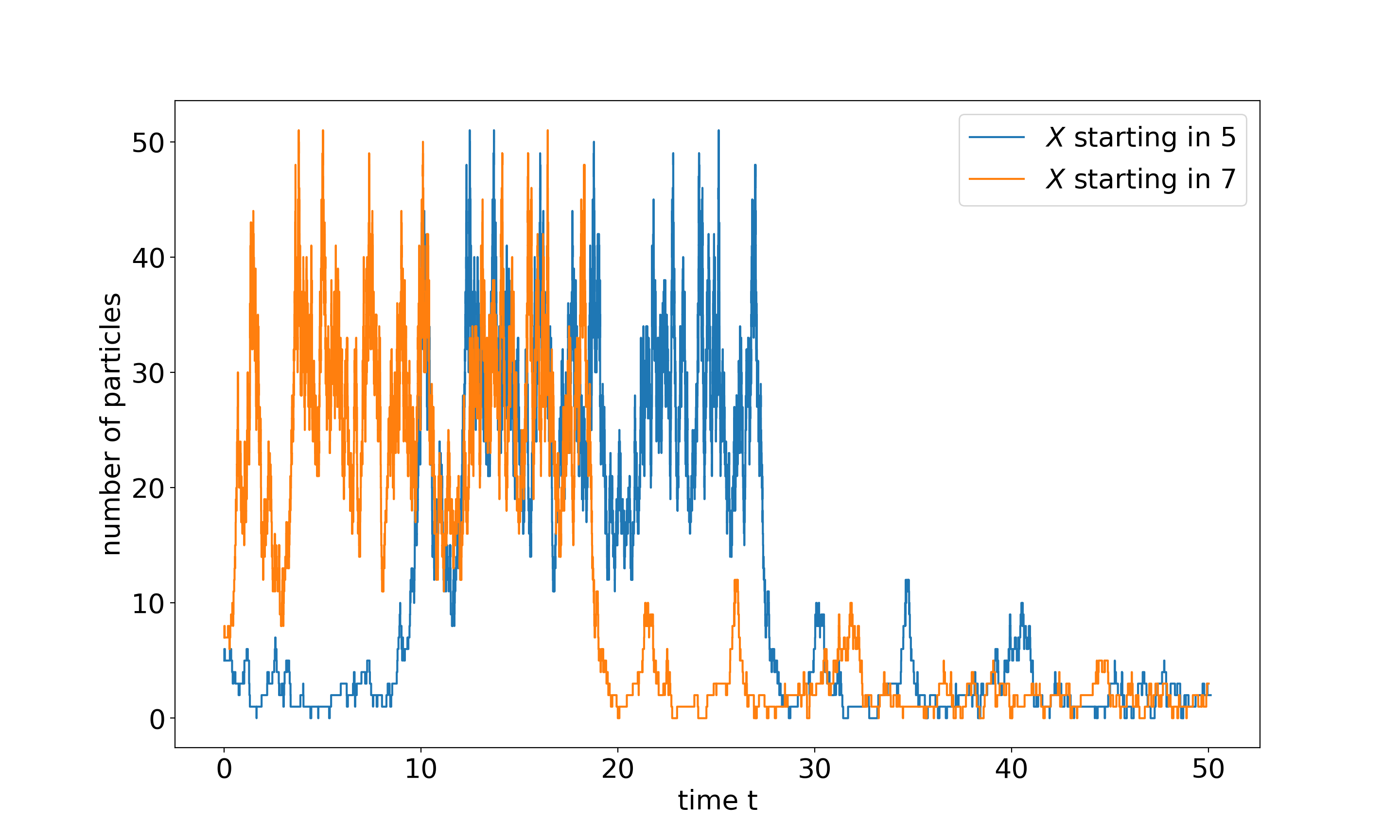##### Time-delayed synchronisation in Markov Jump Processess

A Markov Jump Process formulation is sometimes preferred, when modelling the microscopic interactions of chemical reaction networks. This perspective is somewhat numerically demanding and very often overlooked.

These types of processes exhibit interesting behaviours. In particular, under the same noisy perturbations two different orbits can synchronise with a “time lag”.

We develop theoretical and numerical studies in order to understand delay synchronisation in Markov Jump Processes. These are done in collaboration with Stefanie Winkelmann, Alberto Montefusco, and Nathalie Unger from ZIB.

Please insert any kind of pictures (photos, diagramms, simulations, graphics) related to the project in the above right field (Image with Text), by choosing the green plus image on top of the text editor. (You will be directed to the media library where you can add new files.)
(We need pictures for a lot of purposes in different contexts, like posters, scientific reports, flyers, website,…
Please upload pictures that might be just nice to look at, illustrate, explain or summarize your work.)